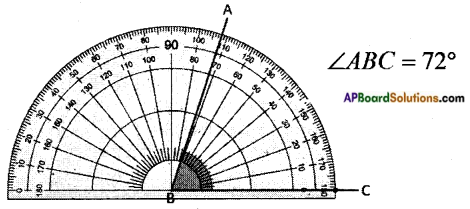Students can go through AP Board 6th Class Maths Notes Chapter 8 Basic Geometric Concepts to understand and remember the concepts easily.

## AP State Board Syllabus 6th Class Maths Notes Chapter 8 Basic Geometric Concepts

→ Line: A line is a straight edge which extends endlessly on both sides. A line has no width.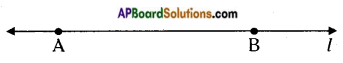Line is a set of infinite points extending in two opposite directions endlessly. A line is represented by any two points lying on it.
The above line is represented by $$\overline{\mathrm{AB}}$$
A line can also be represented by a lower case letter of English alphabet such as l.
A line has no end points.
A line may not be always straight. Sometimes it is curved as shown in the figure.It is called a curved line.→ Ray: A ray is a straight edge starting from a point and extends only in one direction endlessly.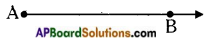A ray has no width.
A ray has only one end point. The end point is called the vertex of the ray. A ray is represented by the initial point and any arbitrary point in the direction in which it extends. Here the ray is $$\overline{\mathrm{AB}}$$.

→ Line segment: A part of a line is called a line segment.
A line segment has two ends.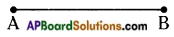A line segment is represented by its two end points. Line segment $$\overline{\mathrm{AB}}$$.
Recall that a small dot made by a shape edged pencil may be treated as a point.

→ History:
Geometry has a long and rich historical nature. The term ‘GEOMETRY’ is derived from the greek word ‘GEOMETRON’. ‘GEO’ means earth and ‘METRON’ means measurement. So, Geometry is the mathematics related to the earth’s measurement.
Early geometry was a collection Of empirically discovered principles concerning lengths, angles, areas and volumes which were developed to meet some practical need in surveying, construction, astronomy and various crafts.
In the ancient India Aryabhatta, Brahmagupta were some of the Indian Mathematicians who contributed their works in geometry.→ Intersecting lines: Two lines meeting at a single point are called intersecting lines. The point is called the point of intersection. Two lines l & m intersecting at the point A.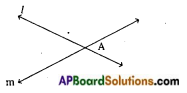→ Concurrent lines: Three or more lines passing through a single point are called concurrent lines and the point is called the point of concurrence.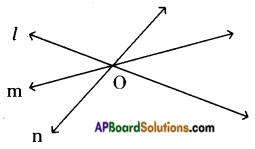Here the lines l, m & n are meeting at a single point O and hence are concurrent lines. The point of concurrence is O.

→ Parallel lines: Two lines are said to be parallel, if they never meet each other.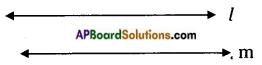The distance between two parallel lines is constant throughout their length.
Here the lines l & m are parallel to one another.

→ Perpendicular lines: Two lines are said to be perpendicular, if they are straight to one another. Two adjacent sides of a paper are perpendicular to each other.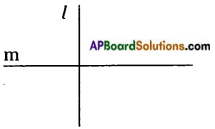→ Measuring the length of a line segment: To measure the length of a given line segment we use a graduated scale or a divider in the instrument box.
Adjust the scale along the line segment and find the readings at the two end points. The difference of readings gives us the length of the line segment.
Adjust the two pointed legs of the divider on the two end points of the given line segment. Now measure the width between the legs on a graduated scale.
Measuring line segments in Centimeters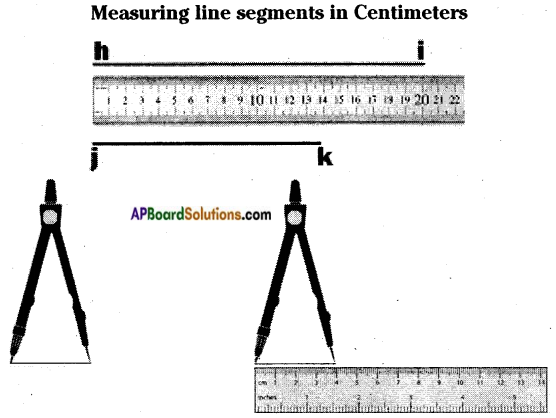→ Angle: A figure formed by two rays with a common end point is called an angle.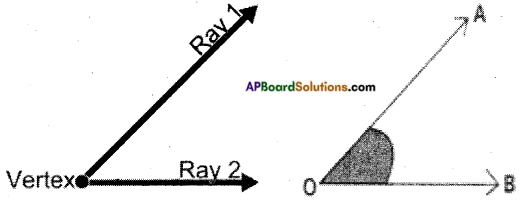The common end point is called the vertex of the angle. The two rays are called the two arms of the angle.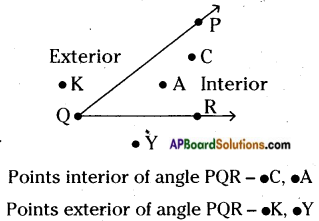→ Sexagesimal system : A system related to number sixty is called sexagesimal system. We use this system in measuring angles.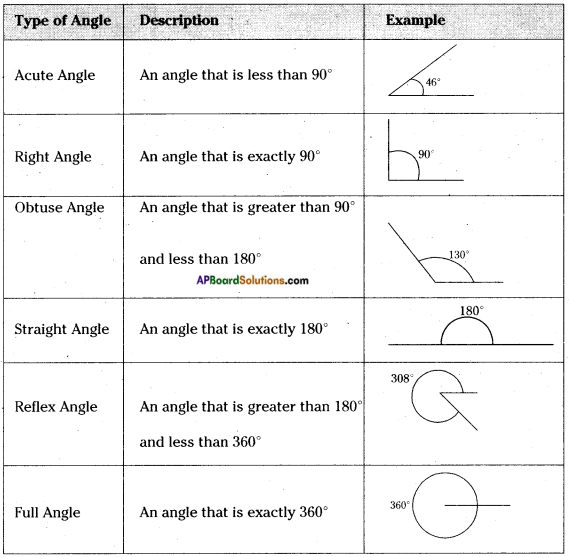Full angle is also called complete angle.
We use protractor to measure the angle.